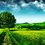# Examination

In an examination, you have to answer 100 questions and for each wrong answer, there will be a cut 0.4 mark from your actual marks.and your results say you get 65 marks, so how many wrong answers do you have?

Note:we get 1 mark for each right answer.Note by Nazmus Sakib
3 years, 7 months ago

This discussion board is a place to discuss our Daily Challenges and the math and science related to those challenges. Explanations are more than just a solution — they should explain the steps and thinking strategies that you used to obtain the solution. Comments should further the discussion of math and science.

When posting on Brilliant:

• Use the emojis to react to an explanation, whether you're congratulating a job well done , or just really confused .
• Ask specific questions about the challenge or the steps in somebody's explanation. Well-posed questions can add a lot to the discussion, but posting "I don't understand!" doesn't help anyone.
• Try to contribute something new to the discussion, whether it is an extension, generalization or other idea related to the challenge.
• Stay on topic — we're all here to learn more about math and science, not to hear about your favorite get-rich-quick scheme or current world events.

MarkdownAppears as
*italics* or _italics_ italics
**bold** or __bold__ bold
- bulleted- list
• bulleted
• list
1. numbered2. list
1. numbered
2. list
Note: you must add a full line of space before and after lists for them to show up correctly
paragraph 1paragraph 2

paragraph 1

paragraph 2

[example link](https://brilliant.org)example link
> This is a quote
This is a quote
    # I indented these lines
# 4 spaces, and now they show
# up as a code block.

print "hello world"
# I indented these lines
# 4 spaces, and now they show
# up as a code block.

print "hello world"
MathAppears as
Remember to wrap math in $$ ... $$ or $ ... $ to ensure proper formatting.
2 \times 3 $2 \times 3$
2^{34} $2^{34}$
a_{i-1} $a_{i-1}$
\frac{2}{3} $\frac{2}{3}$
\sqrt{2} $\sqrt{2}$
\sum_{i=1}^3 $\sum_{i=1}^3$
\sin \theta $\sin \theta$
\boxed{123} $\boxed{123}$

## Comments

Sort by:

Top Newest

Assuming we get 1 mark for each right answer before deduction, and let the number of questions wrongly answered be $x$. Then we have

\begin{aligned} (100-x)-0.4x & =65 \\ 1.4x & = 35 \\ \implies x & = 25 \end{aligned}

- 3 years, 7 months ago

Log in to reply

@Chew-Seong Cheong Thanks a lot,now I understand it and If we say "we get 2 marks for each right answer" then the result of the number of questions wrongly answered will stay the same?I think the mark for each right answer is not necessary to know to get the amount of wrong answers

- 3 years, 7 months ago

Log in to reply

200-x-0.4x=65, 135=1.4x. x is not a whole number, meaning that x does matter

- 3 years, 7 months ago

Log in to reply

For 2 marks for each right answer, 200 is marks not the amount of question.and what do you mean by $x$? amount of right answers or amount of wrong answers?

- 3 years, 7 months ago

Log in to reply

It should be $2(100-x)-0.4x = 65$. Then $x=56/25$

- 3 years, 7 months ago

Log in to reply

I mean $x=135/2.4=56.25$

- 3 years, 7 months ago

Log in to reply

@Chew-Seong Cheong so is it necessary to know the mark for each right answer to get the amount of wrong answers?

- 3 years, 7 months ago

Log in to reply

No, if it is two marks there is no integer solution. We cannot have 56.25 wrong answers, right? Obviously, the right mark cannot be lower than 0.4. You must understand the equation instead of guessing.

- 3 years, 7 months ago

Log in to reply

What is the mark for each correct answer?

- 3 years, 7 months ago

Log in to reply

I've edited that "we get 1 mark for each right answer." although I think the mark for each right answer is not necessary to know to get the amount of wrong answers.

- 3 years, 7 months ago

Log in to reply

- 3 years, 7 months ago

Log in to reply

Staff - 3 years, 7 months ago

Log in to reply

I was stuck at the beginning,didn't know how to start.

- 3 years, 7 months ago

Log in to reply

×

Problem Loading...

Note Loading...

Set Loading...